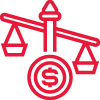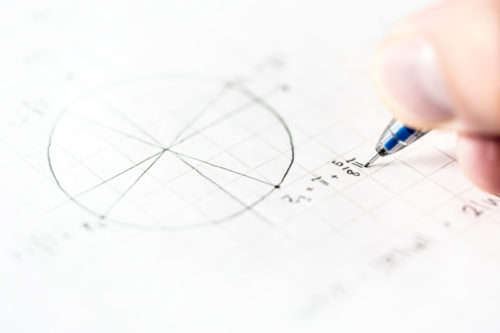# A Math Tuition

### Sec 3 | Sec 4 | Sec 5 O  Level | N Level A Math Tuition

Additional Mathematics (A Math) is a core subject that facilitates the learning of the higher level Math modules in the tertiary courses (poly or JC etc). Without A Math, some courses will be out of bounds for the students and some Math modules will be too abstract to understand.

In the N level and O level syllabus, A Math involves a huge application of algebra knowledge (mastered in Sec 1 and Sec 2) on the new topics in Sec 3 and Sec 4. While there are more formulas in A Math than E Math, the solutions in A Math are often long and tedious. It also requires the students to develop a deep understanding of the concepts as well as acute analysis of the questions.

Einstein A Math curriculum is meticulously crafted to sharpen the students’ understanding on the topics. Einstein champions consistency and active learning in the students, equip them with the skills and wits to carve out their edge in A Math.

### Program HighlightsMagnify concepts
– Direct and simplified explanations
– Internalise concepts effectively
– Cross link different concepts confidentlyDetailed coverage
– Exam based questions
– Safe and effective method
– Step by step approachDevelop mathematical competence
– Mathematical reasoning skills
– In-depth analytical skills
– Zero error perfection skills

## Einstein A Math MentorsEric Lim (Mr Lim)
Chief Educator
Master trainer (teaching)
NUS Bachelor of Science
(Major in Pure Math)Serena
Super Educator

NUS Bachelor of Engineering (Honours)
(Major in Civil Engineering)Azhar
Senior Educator
Senior Trainer (Teaching)

NUS Bachelor of Science (Honours)
(Double Major in Physics and Pure Math)

Prepare for your major exams with Einstein. For 15 years, we have helped students of different ability levels to score in the N Levels and O Levels. Our teaching methodology has proven to be a favourite with our students – super clear, systematic and successful.

### Star Features Of Our A Math Classes##### Small class size

1 to 9 students##### Flat Monthly Fees

4 weeks or 5 weeks##### Unbeatable Price

S3: \$160 | S4: \$180

No Deposit. No collection of lump sum fees.

2 x 1.5h

Weekly

2.5h

Weekly

1.5h

Weekly

1.5h

Weekly

### A Math Program Coverage##### Indices and Surds
• Apply algebra concepts to simplify indices expressions and express values in surds form.
• Solve indices equation and surds equations step by step using the most effective method.
• Recognise the different forms of quadratic inequality and use the right method to solve.
• Understand the deeper concepts and master the various methods to solve common exam questions with all the steps clearly shown.
##### Remainder and Factor Theorem
• Solve different types of identities effectively by identifying the correct method to apply.
• To find the remainder when the original expression is divided by a linear factor or a quadratic factor.
• Solve algebra equations of higher orders of x (and above) by using factor theorem.  Factorise the expression using long division accurately.
• Compare 2 equations and observe a link between them and identify the correct transformation that will map 1 equation onto another equation by replacing x with another variable.
##### Trigo and Further Trigo
• Memorise the values of the trigo for special angles (both radian and degree) in surds form.
• Identify the quadrants correctly and solve trigo equations to work out all the angles within the given range.
• Prove trigo identities using the correct formulas.
• Sketch trigo curves accurately by working out the amplitude and period of 1 cycle.
• Solve further trigo problem sums using the R-formula.
##### Coordinates Geometry and Circles
• Find equations of straight lines including parallel lines, perpendicular lines and perpendicular bisectors using gradient and a pair of coordinates.
• Calculate the area of triangle or quadrilateral using the shoe-lace method.
• Draw out the perpendicular distance from a point to a line and formulate an equation using the area of triangle to solve for the perpendicular distance.
• Use ratios to find the coordinates of a point.
• Identify the radius and centre to find the equation of a circle.
• Memorise and apply the formulas to find the radius and the centre of a circle.
• Work out the coordinates of the centre of a circle under reflection and its radius when the circle is tangent to a straight line.
##### Linear Law and Absolute Functions
• Solve modulus equations correctly and identify the values to reject with reasonings.
• Draw the graphs of absolute functions using step by step approach and identify the range and state the number of solutions between the absolute function and another line.
• Simplify expressions with modulus signs correctly.
• Draw out the best fit line and solve for the gradient and unknown variables in the equation.
• Simplify a complicated equation to make y the subject.
##### Binomial Theorem
• Apply the formula to expand , n > 2.  Simplify the terms in the expansion correctly.
• Apply the formula of the  term to represent a particular term such as the term of independent of x and solve for the unknown variable.
• Use the expanded terms to find the numerical value of an expression by substituting x with a number.
##### Differentiation
•  Master the skills of differentiating constant, x, , and  confidently.
• Visualise the tangents and normals and work out the equations correctly.
• Apply the chain rule accurately to calculate the rate of change.
• Find the stationary points and use the 2nd derivative to determine the nature.
• Understand the maximum/minimum problem sum and form the right equation to solve for the unknow variable.
• Sketch the curve accurately by marking out the x-intercept, y-intercept and the stationary points.
• Solve differentiation questions involving trigo, ln and  by applying the correct formulas.
##### Integration
• Integrate  to find the original equation of the curve.
• Use definite integrals to calculate the areas under the curve with respect to the x-axis and y-axis.
• Combine differentiation and integration to solve problem sums including trigo, ln and  confidently.
##### Kinematics
• Formulate acceleration, speed and displacement equations using differentiation and integration techniques.
• Construct displacement diagrams accurately showing the path of the travelling object clearly indicating the turning points and the distance travelled.
##### Plane Geometry
• Use concepts of similar triangles, congruent triangles, angle properties of circle and plane geometry formulas to prove a statement.